## PagesPi Cubed: \$9.99visual math application designed from the ground up for a touch-based interface. Unlike traditional calculators, Pi Cubed lets you construct, typeset, and instantly evaluate mathematical expressions using an interactive menu system.Quick Graph : FREEThis is a powerful graphic calculator that takes full advantage of the multitouch display and the powerful graphic capabilities of the iPad and iPhone, both in 2D and 3D. A simple, yet intuitive interface that makes it easy to enter and edit equations and visualize them.Equation Genius: FREEEquation Genius will help you do you math in seconds. It supports: 1st degree equations (ax + b = c), Quadratic (2nd degree) equations (ax^2 + bx + c = 0), Cubic (3rd degree) equations (ax^3 + bx^2 + cx + d = 0), System of two unknowns (2 variables), System of three unknowns (3 variables).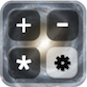MyCalculator \$1.99A scientific calculator for iPad and iPhone that solves as you type! MyCalculator also features an innovative memory system to store and recall answers. Simply touch answers to store them in multiple memory slots.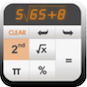Scientific Calculator: \$ 1.99Solve is an Touch calculator replacement designed with simplicity, usability, and beauty in mind...Once you try Solve you never go back. Access to 4 different calculators: 1- Handwriting calculator 2- Scientific calculator, 3- Quadratic equation solver 4- Linear equations.Maths Ref: \$2.99With over 250 equations it will be tough to find the one you need. In 3.1, we added super smart search bar to aid your searching. Just pull down the list to reveal the bar and start typing. If you have sinh() in mind, type sinh or hyperbolic. You will be amazed how useful it is. iFormulas: FREEis a clean, simple, easy to use mathematical formula application. Provides basics to survive your Algebra, Calculus, Geometry or Trigonometry class. It does not give you answers but provides an easy navigational guide. Over 330 different formulas, definitions, laws, properties, etc.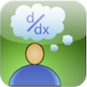Formulus: \$1.99A perfect study tool. It is a simple, easy to use, easy to navigate collection of the most important formulas and topics for high school and college students taking Calculus and Differential Equations.Equation Solver: FREEMath Homework Solver (MHS) is making math easy. Get solutions to dozens of problems in various topics such as: Arithmetic or Geometric sequence, Line problems, Equations, Proportions, Percentages, Complex Numbers, Inequalities, Vectors etc,Fractions Calculator: \$ 0.99Just type in the fractions you want using the keypad. Select any of the editable fields and the keypad appears. The field being editing is shown with a yellow background. You can change more than one number at a time by selecting each field and typing the number. When you have set the fractions as you want then select 'OK'.DiaMath: \$0.99 configurable to quiz you with 10, 20 or 30 problems. All the problems are generated by the iPhone and randomized so there is a virtual unlimited set of possibilities. DiaMath provides three different levels of difficulty as well as allowing you to choose a range of numbers from 1 to 99. Additionally, DiaMath has a timer.Talking Calculator: \$0.99Hear all of your numbers and results announced as they appear on the screen! Great for visually impaired or for anyone who wants to verify accuracy without looking back at the screen. Also a wonderful educational app for children who are learning math because it gives them audible reinforcement.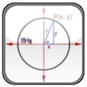Trigonometry Calculator: \$2.99Interactive Trigonometry Calculator contains all Trigonometry functions and formulas. Users can solve any triangles, circles and unit circles problems very easily using this calculator. No more trying to grab your calculator or trying to recall the sin, cos & tan rules. This is the only trigonometry calculator you will ever need.Mathsmagic: \$0.99Amaze and delight others as you multiply, divide, and square at lightning fast speed. Learn and practice the tricks of mental math calculation in a fun and engaging application. Study any of the math tricks and then practice them as you progress through various levels of proficiency.Maths Plus: \$0.99by presenting you with randomly selected simple maths problems that require combining basic arithmetic knowledge, helps you to develop your problem solving skills, and by allowing you to play with others, it adds a level of fun and challenge to this process.TanZen: \$1.99Tangrams have been challenging minds for centuries, with the deceptively simple goal of combining seven geometric pieces into a shape. Choose a puzzle to solve, and try to fit all seven game pieces within the shaded puzzle area without overlapping. TanZen will recognize when the puzzle is finished.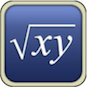Symbolic Calculator: \$0.99With full-featured algebra capabilites on par with high-end scientific calculators this applications lets you perform all kind of different operations from the simplest multiplications to computing the integrals of the most complicated functions.Alegbra Solver: \$0.99This program is NOT just a flash card application. Algebra Solver SOLVES math formulas and equations; after you solve them, you can even EMAIL them to others using the built-in email button. From simple formulas, to more complex physics problems,.My Formulas: \$0.99Fully customizable formula calculator. Keeping ease of use in mind, myFormulas provides a new and intuitive way of creating, editing and calculating diverse formulas. You can make a wide variety of formulas which can be as simple as a tip calculator and a unit converter, or as sophisticated as an electronic engineering calculator.The Mathmaster: \$0.99Need more help with your math facts? Chances are yes, and that's exactly why there is MathMaster. MathMaster also includes a powerful settings page allowing you to fully customize which problems it generates. MathMaster supports a variety of mathematical operations. LIST OF UNREVIEWED MATHS APPS  3D Plotter XL 4D Spin 600 Formulas Abakus AceKids Maths Game HD Algebra Champ Algebra Helper Algebra Pro Algebra Touch All-in-One Maths Alien Equation Angles Calculator Arithmetric Arithfit Basic maths Calc It Calculus Pro Calculator XL Calculator AXL Calculator LCD HD PRO Calcbot CalCul CalcTraining Converter for iPad Conversion Calculator Couch Calculator Digits Calculator for iPad Dimension Calculator Dr Pi Easel Algebra eSolver Equation Equations Everyday Mathematics Fastcalc Flash Maths FlowMath Formula Pro Fractions App Fractals Fractional Editor Free Graphic Calculator Fractions Helper fx Pad fx Integer Geometry Geometry Combat Geometry Wars Geometry Stash Globe Convert Grafly Graph It Graphing Calculator Graphing Calculator PLUS Graphulator Gravity GroupCalc HiCalc iArithmetric iAttractor iFractual iFactor iFactor Quadratics Interactive Trigonometry Calculator Integral Calculator Intro to Maths iMath iMaths iMathsLab iMathematica iMathematics 9 in 1 iMeasure IQ Gym iTrig Lemonade Stand Long Division MaCalc MandelBrot MathBoard Math Bingo Maths Cards Math Drills MathGirl Number Garden Math Magic Math Ninja HD Mathematical Formulas Mathination Mathomatic Maths Equation Solver Maths Flash Cards Maths Formulas Maths Homework Solver Maths Lab Maths PRO Math Ref Maths Trivia Quiz Maths Tricks MeStudying Algebra MightyMaths Motion Maths Multimeasures HD MyAttractor NineGaps - Puzzle Game NoteCalc Number Line Oxford Dictionary of Mathematics P183 Graphing Calculator Pad Maths PCalc Lite Pi Cubed Polar Sweep Polysolve PopMaths PopMaths Basic Maths Powerone Calculator Probability PRO Quadratic Master Quadratic Equation Solver Quick Multiplication Quick Protactor Rec Polar Note Calc HD Rocket Maths Ruler Scientific Calculator Shady Puzzles Smart Convertor Smart Maths Solve24 SpaceTime Sumstacker Statistics 1 Statistic Toolkit Statistics Visualiser StatsMate Talkulator TallyZoo Timestables TouchPlot Touchy Math Trade First Subtraction Trigger UslideRule Vectorama Vector Calculator Writeanswer Wolfram Algebra Course